# Basics Of Options Trading Explained

Compiled by Rekhit Pachanekar

Before we delve deep into the world of options trading, let’s take a moment to understand why do we need options at all. If you are thinking it is just another way to make money and was created by some fancy guys in suits working in Wall Street, well, you are wrong. The options world predates the modern stock exchanges by a large margin.

While some credit the Samurai for giving us the foundation on which options contracts were based, some actually acknowledge the Greeks for giving us an idea on how to speculate on a commodity, in this case, the harvest of olives. In both cases, humans were trying to guess the price of a food item and trade accordingly (rice in the case of samurais), long before the modern world put in various rules and set up exchanges.

With this in mind, let us try to answer the first question in your mind.

Let’s take a very simple example to understand options trading. Consider that you are buying a stock for Rs. 3000. But the broker tells you about an exciting offer, that you can buy it now for Rs. 3000 or you can give a token amount of Rs. 30 and reserve the right to buy it at Rs. 3000 after a month, even if the stock increases in value at that time. But that token amount is non-refundable!

You realise that there is a high chance that the stock would cross Rs. 3030 and thus, you can breakeven at least. Since you have to pay only Rs. 30 now, the remaining amount can be used elsewhere for a month. You wait for a month and then look at the stock price.

Now, depending on the stock price, you have the option to buy the stock from the broker or not. Of course, this is an over-simplification but this is options trading in a gist.In the world of trading, options are instruments that belong to the derivatives family, which means its price is derived from something else, mostly stocks. The price of an option is intrinsically linked to the price of the underlying stock.

A formal definition is given below:
A stock option is a contract between two parties in which the stock option buyer (holder) purchases the right (but not the obligation) to buy/sell shares of an underlying stock at a predetermined price from/to the option seller (writer) within a fixed period of time.

We are going to make sure that by the end of this article you are well versed with the options trading world along with trying out a few options trading strategies as well. We will cover the following points in this article. If you feel that you want to skip the basics of options, then head straight to the options trading strategies

Let’s start now, shall we!

There must be a doubt in your mind that why do we even have options trading if it is just another way of trading. Well, here are a few points which make it different from trading stocks

• The Options contract has an expiration date, unlike stocks. The expiration can vary from weeks, months to years depending upon the regulations and the type of Options that you are practising. Stocks, on the other hand, do not have an expiration date.
• Unlike Stocks, Options derive their value from something else and that’s why they fall under the derivatives category
• Options are not definite by numbers like Stocks
• Options owners have no right (voting or dividend) in a company unlike Stock owners

It is quite often that some people find the Option’s concept difficult to understand though they have already followed it in their other transactions, for e.g. car insurance or mortgages.  In this part of the article, we will take you through some of the most important aspects of Options trading before we get down to the world of options trading.

## Options terminologies

### Strike Price

The Strike Price is the price at which the underlying stocks can be bought or sold as per the contract. In options trading, the Strike Price for a Call Option indicates the price at which the Stock can be bought (on or before its expiration) and for Put Options trading it refers to the price at which the seller can exercise its right to sell the underlying stocks (on or before its expiration)

Since the Options themselves don’t have an underlying value, the Options premium is the price that you have to pay in order to purchase an Option. The premium is determined by multiple factors including the underlying stock price, volatility in the market and the days until the Option’s expiration. In options trading, choosing the premium is one of the most important components.

### Underlying Asset

In options trading, the underlying asset can be stocks, futures, index, commodity or currency. The price of Options is derived from its underlying asset. For the purpose of this article, we will be considering the underlying asset as the stock. The Option of stock gives the right to buy or sell the stock at a specific price and date to the holder. Hence its all about the underlying asset or stocks when it comes to Stock in Options Trading.

### Expiration Date

In options trading, all stock options have an expiration date. The expiration date is also the last date on which the Options holder can exercise the right to buy or sell the Options that are in holding. In Options Trading, the expiration of Options can vary from weeks to months to years depending upon the market and the regulations.

### Options Style

There are two major types of Options that are practised in most of the options trading markets.

• American Options which can be exercised anytime before its expiration date
• European Options which can only be exercised on the day of its expiration

### Moneyness (ITM, OTM & ATM)

It is very important to understand the Options Moneyness before you start trading in Stock Options. A lot of options trading strategies are played around the Moneyness of an Option.

It basically defines the relationship between the strike price of an Option and the current price of the underlying Stocks. We will examine each term in detail below.

#### When is an Option in-the-money?

• Call Option - when the underlying stock price is higher than the strike price
• Put Option - when the underlying stock price is lower than the strike price

#### When is an Option out-of-the-money?

• Call Option - when the underlying stock price is lower than the strike price
• Put Option - when the underlying stock price is higher than the strike price

#### When is an Option at-the-money?

• When the underlying stock price is equal to the strike price.
Take a break here to ponder over the different terms as we will find it extremely useful later when we go through the types of options as well as a few options trading strategies.

## Type of options

In the true sense, there are only two types of Options i.e Call & Put Options. We will understand them in more detail.

### To Call or Put

A Call Option is an option to buy an underlying Stock on or before its expiration date. At the time of buying a Call Option, you pay a certain amount of premium to the seller which grants you the right (but not the obligation) to buy the underlying stock at a specified price (strike price).

Purchasing a call option means that you are bullish about the market and hoping that the price of the underlying stock may go up. In order for you to make a profit, the price of the stock should go higher than the strike price plus the premium of the call option that you have purchased before or at the time of its expiration.

In contrast, a Put Option is an option to sell an underlying Stock on or before its expiration date. Purchasing a Put Option means that you are bearish about the market and hoping that the price of the underlying stock may go down. In order for you to make a profit, the price of the stock should go down from the strike price plus the premium of the Put Option that you have purchased before or at the time of its expiration.

In this manner, both Put and Call option buyer’s loss is limited to the premium paid but profit is unlimited. The above explanations were from the buyer's point of view. We will now understand the put-call options from the seller’s point of view, ie options writers. The Put option seller, in return for the premium charged, is obligated to buy the underlying asset at the strike price.

Similarly, the Call option seller, in return for the premium charged, is obligated to sell the underlying asset at the strike price. Is there a way to visualise the potential profit/loss of an option buyer or seller? Actually, there is. An option payoff diagram is a graphical representation of the net Profit/Loss made by the option buyers and sellers.

Before we go through the diagrams, let’s understand what the four terms mean. As we know that going short means selling and going long means buying the asset, the same principle applies to options. Keeping this in mind, we will go through the four terms.

• Short call - Here we are betting that the prices will fall and hence, a short call means you are selling calls.
• Short put - Here the short put means we are selling a put option
• Long call - it means that we are buying a call option since we are optimistic about the underlying asset’s share price
• Long put - Here we are buying a put option.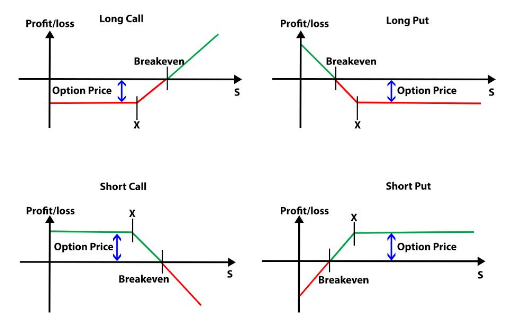where,

S = Underlying Price
X = Strike Price

Break-even point is that point at which you make no profit or no loss.

The long call holder makes a profit equal to the stock price at expiration minus strike price minus premium if the option is in the money. Call option holder makes a loss equal to the amount of premium if the option expires out of money and the writer of the option makes a flat profit equal to the option premium.

Similarly, for the put option buyer, profit is made when the option is in the money and is equal to the strike price minus the stock price at expiration minus premium. And, the put writer makes a profit equal to the premium for the option.

All right, until now we have been going through a lot of theory. Let’s switch gears for a minute and come to the real world. How do options look like? Well, let’s find out.

### What does an options trading quote consist of?

If you were to look for an options quote on Apple stock, it would look something like this: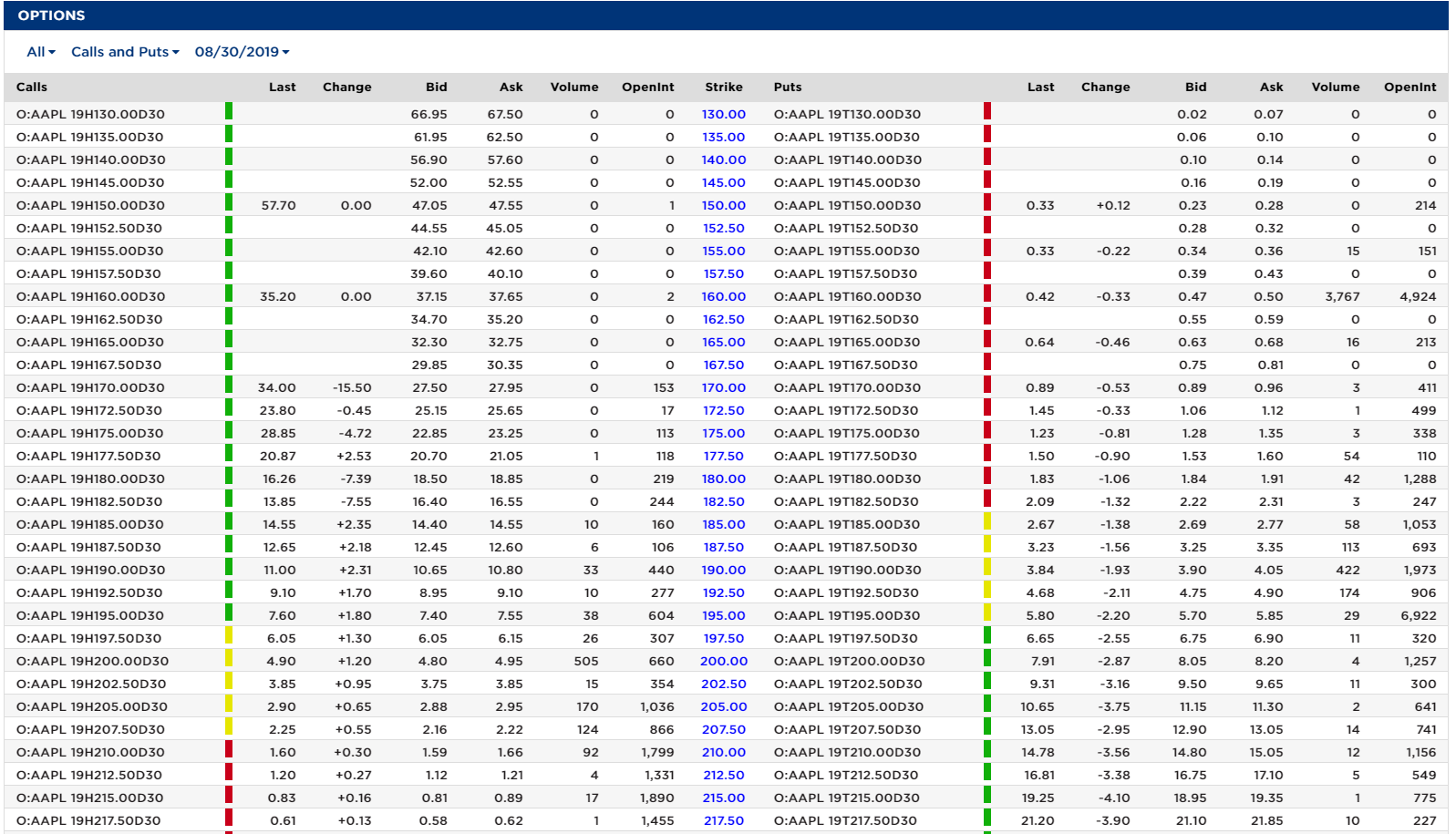When this was recorded, the stock price of Apple Inc. was \$196. Now let’s take one line from the list and break it down further.

Eg.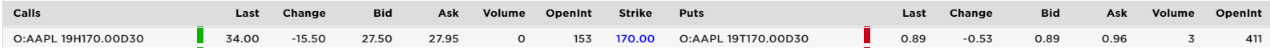In a typical options chain, you will have a list of call and put options with different strike prices and corresponding premiums. The call option details are on the left and the put option details are on the right with the strike price in the middle.

• The symbol and option number is the first column.
• The “last” column signifies the amount at which the last time the option was bought.
• Change” indicates the variance between the last two trades of the said options
• Bid” column indicates the bid submitted for the option.
• Volume” indicates the number of options traded. Here the volume is 0.
• Open Interest” indicates the number of options which can be bought for that strike price.

The columns are the same for the put options as well. In some cases, the data provider signifies whether the option is in the money, at the money or out of money as well. Of course, we need an example to really help our understanding of options trading. Thus, let’s go through one now.

We will go through two cases to better understand the call and put options.

For simplicity’s sake, let us assume the following:

• Price of Stock when the option is written: \$100
• Expiration date: 1 month after the option is bought

### Case 1:

The current price of stock: \$110. Strike price: \$120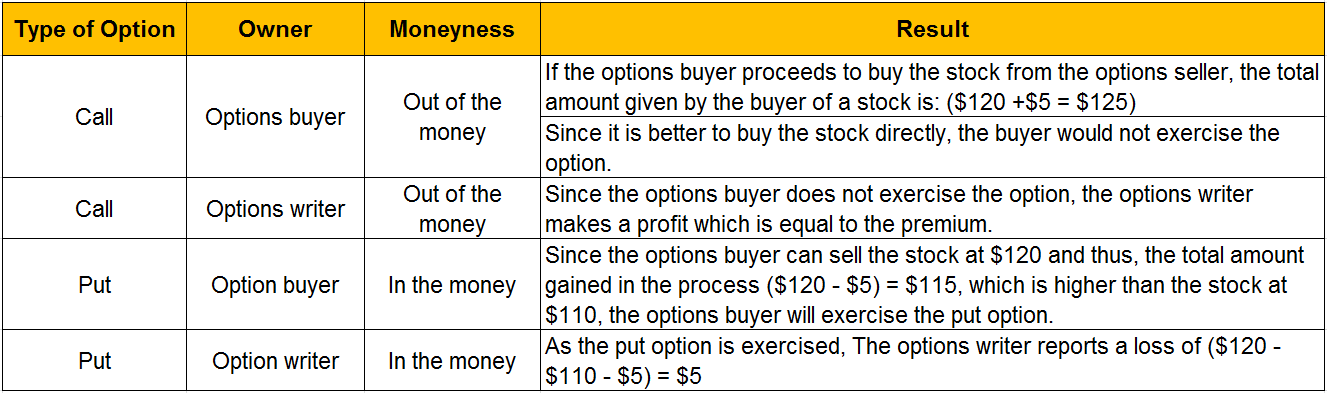### Case 2:

The current price of stock: \$120. Strike price: \$110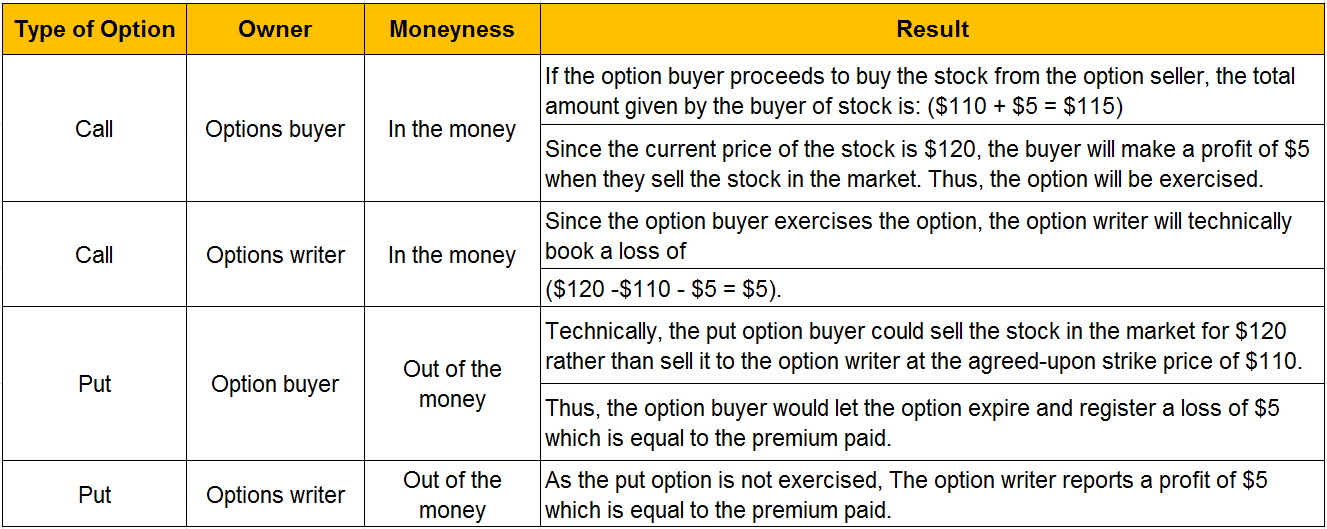Considering that we have gone through the detailed scenario of each option, how about we combine a few options together. Let’s understand an important concept which many professionals use in options trading.

## What is Put-Call Parity In Python?

Put-call parity is a concept that anyone who is interested in options trading needs to understand. By gaining an understanding of put-call parity you can understand how the value of call option, put option and the stock are related to each other. This enables you to create other synthetic position using various option and stock combination.

### The principle of put-call parity

Put-call parity principle defines the relationship between the price of a European Put option and European Call option, both having the same underlying asset, strike price and expiration date. If there is a deviation from put-call parity, then it would result in an arbitrage opportunity. Traders would take advantage of this opportunity to make riskless profits till the time the put-call parity is established again.

The put-call parity principle can be used to validate an option pricing model. If the option prices as computed by the model violate the put-call parity rule, such a model can be considered to be incorrect.

### Understanding Put-Call Parity

To understand put-call parity, consider a portfolio “A” comprising of a call option and cash. The amount of cash held equals the call strike price. Consider another portfolio “B” comprising of a put option and the underlying asset.

S0 is the initial price of the underlying asset and ST is its price at expiration.
Let “r” be the risk-free rate and “T” be the time for expiration.
In time “T” the Zero-coupon bond will be worth K (strike price) given the risk-free rate of “r”.

Portfolio A = Call option + Zero-coupon bond

Portfolio B = Put option + Underlying Asset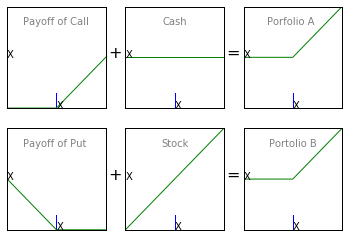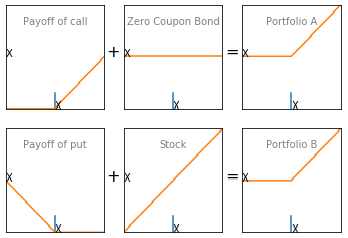If the share price is higher than X the call option will be exercised. Else, cash will be retained. Hence, at “T” portfolio A’s worth will be given by max(ST, X).

If the share price is lower than X, the put option will be exercised. Else, the underlying asset will be retained. Hence, at “T”, portfolio B’s worth will be given by max (ST, X).

If the two portfolios are equal at time, “T”, then they should be equal at any time. This gives us the put-call parity equation.

Equation for put-call parity:

C + Xe-rT = P + S0

In this equation,

• C is the premium on European Call Option
• P is the premium of European Put Option
• S0 is the spot price of the underlying stock
• And, Xe-rT is the current value (discounted value) of Zero-coupon bond (X)

We can summarize the payoffs of both the portfolios under different conditions as shown in the table below.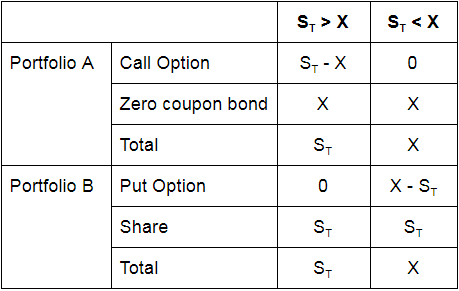From the above table, we can see that under both scenarios, the payoffs from both the portfolios are equal.

### Required Conditions For Put-call Parity

For put-call parity to hold, the following conditions should be met. However, in the real world, they hardly hold true and put-call parity equation may need some modifications accordingly. For the purpose of this blog, we have assumed that these conditions are met.

• The underlying stock doesn’t pay any dividend during the life of the European options
• There are no transaction costs
• There are no taxes
• Shorting is allowed and there are no borrow charges

Hence, put-call parity will hold in a frictionless market with the underlying stock paying no dividends.

### Arbitrage Opportunity

In options trading, when the put-call parity principle gets violated, traders will try to take advantage of the arbitrage opportunity. An arbitrage trader will go long on the undervalued portfolio and short the overvalued portfolio to make a risk-free profit.

#### How to take advantage of arbitrage opportunity

Let us now consider an example with some numbers to see how trade can take advantage of arbitrage opportunities. Let’s assume that the spot price of a stock is \$31, the risk-free interest rate is 10% per annum, the premium on three-month European call and put are \$3 and \$2.25 respectively and the exercise price is \$30.

In this case, the value of portfolio A will be,

C+Xe-rT = 3+30e-0.1 * 3/12 = \$32.26

The value of portfolio B will be,

P + S0 = 2.25 + 31 = \$33.25

Portfolio B is overvalued and hence an arbitrageur can earn by going long on portfolio A and short on portfolio B. The following steps can be followed to earn arbitrage profits.

• Short the stock. This will generate a cash inflow of \$31.
• Short the put option. This will generate a cash inflow of \$2.25.
• Purchase the call option. This will generate cash outflow of \$3.
• Total cash inflow is -3 + 2.25 + 31 = \$30.25.
• Invest \$30.25 in a zero-coupon bond with 3 months maturity with a yield of 10% per annum.

Return from the zero coupon bond after three months will be 30.25e 0.1 * 3/12 = \$31.02.

If the stock price at maturity is above \$30, the call option will be exercised and if the stock price is less than \$30, the put option will be exercised. In both the scenarios, the arbitrageur will buy one stock at \$30. This stock will be used to cover the short.

Total profit from the arbitrage = \$31.02 - \$30 = \$1.02

Well, shouldn’t we look at some codes now?

### Python Codes Used For Plotting The Charts

The below code can be used to plot the payoffs of the portfolios.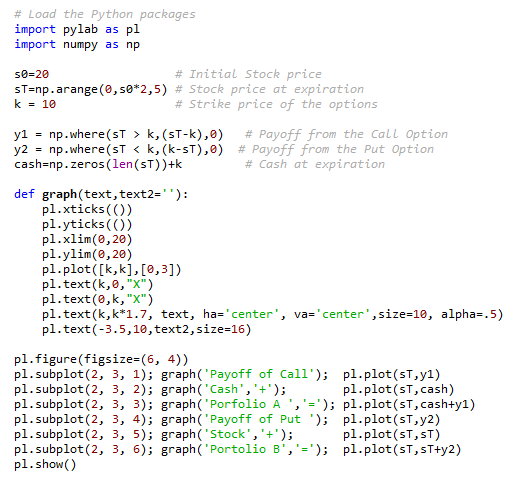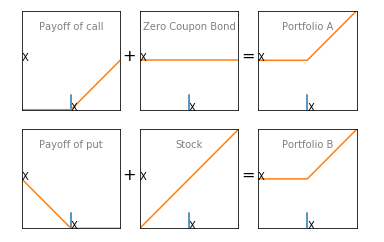So far, we have gone through the basic concepts in options trading and looked at an options trading strategy as well. At this juncture, let’s look at the world of options trading and try to answer a simple question.

### Why is Options Trading attractive?

Options are attractive instruments to trade in because of the higher returns. An option gives the right to the holder to do something, with the ‘option’ of not to exercise that right. This way, the holder can restrict his losses and multiply his returns.

While it is true that one options contract is for 100 shares, it is thus less risky to pay the premium and not risk the total amount which would have to be used if we had bought the shares instead. Thus your risk exposure is significantly reduced.However, in reality, options trading is very complex and that is because options pricing models are quite mathematical and complex.

So, how can you evaluate if the option is really worth buying? Let’s find out.

The key requirement in successful options trading strategies involves understanding and implementing options pricing models. In this section, we will get a brief understanding of Greeks in options which will help in creating and understanding the pricing models.

#### Options Pricing

Options Pricing is based on two types of values

##### Intrinsic Value of an option

Recall the moneyness concept that we had gone through a few sections ago. When the call option stock price is above the strike price or when put option stock price is below the strike price, the option is said to be “In-The-Money (ITM)”, i.e. it has an intrinsic value. On the other hand, “Out of the money (OTM)” options have no intrinsic value. For OTM call options, the stock price is below the strike price and for OTM put options; stock price is above the strike price. The price of these options consists entirely of time value.

##### Time Value of an option

If you subtract the amount of intrinsic value from an options price, you’re left with the time value. It is based on the time to expiration. You can enroll for this free online options trading python course on Quantra and understand basic terminologies and concepts that will help you in options trading. We know what is intrinsic and the time value of an option. We even looked at the moneyness of an option. But how do we know that one option is better than the other, and how to measure the changes in option pricing. Well, let’s take the help of the greeks then.

## Options Greeks

Greeks are the risk measures associated with various positions in options trading. The common ones are delta, gamma, theta and vega. With the change in prices or volatility of the underlying stock, you need to know how your options pricing would be affected. Greeks in options help us understand how the various factors such as prices, time to expiry, volatility affect the options pricing.

Delta measures the sensitivity of an option’s price to a change in the price of the underlying stock. Simply put, delta is that options greek which tells you how much money a stock option will rise or drop in value with a \$1 rise or drop in the underlying stock. Delta is dependent on underlying price, time to expiry and volatility. While the formula for calculating delta is on the basis of the Black-Scholes option pricing model, we can write it simply as,

Delta = [Expected change in Premium] / [Change in the price of the underlying stock]

Let’s understand this with an example for a call option:
We will create a table of historical prices to use as sample data. Let’s assume that the option will expire on 5th March and the strike price agreed upon is \$140.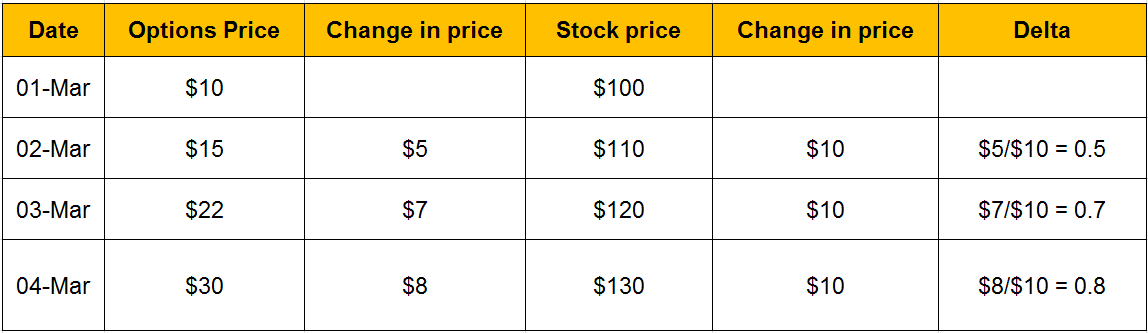Thus, if we had to calculate the delta for the option on 2nd March, it would be \$5/\$10 = 0.5.

Here, we should add that since an option derives its value from the underlying stock, the delta option value will be between 0 and 1. Usually, the delta options creeps towards 1 as the option moves towards “in-the-money”.

While the delta for a call option increases as the price increases, it is the inverse for a put option. Think about it, as the stock price approaches the strike price, the value of the option would decrease. Thus, the delta put option is always ranging between -0 and 1.

Gamma measures the exposure of the options delta to the movement of the underlying stock price. Just like delta is the rate of change of options price with respect to underlying stock’s price; gamma is the rate of change of delta with respect to underlying stock’s price. Hence, gamma is called the second-order derivative.

Gamma = [Change in an options delta] / [Unit change in price of underlying asset]

Let's see an example of how delta changes with respect to Gamma. Consider a call option of stock at a strike price of \$300 for a premium of \$15.

• Strike price: \$300
• Initial Stock price: \$150
• Delta: 0.2
• Gamma: 0.005
• New stock price: \$180
• Change in stock price: \$180 - \$150 = \$30

Now,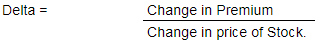Thus, Change in Premium = Delta * Change in price of stock = 0.2 * 30 = 6.
Thus, new premium = \$15 + \$6 = \$21

Change in delta = Gamma * Change in stock price = 0.005 * 30 = 0.15
Thus, new delta = 0.2 + 0.15 = 0.35.

Let us take things a step further and assume the stock price increases another 30 points, to \$210.

Now,
New stock price: \$210
Change in stock price: \$210 - \$180 = \$30

Now,Change in premium = Delta *Change in 0.35*30 = \$10.5
Thus, new premium = \$21 + \$10.5 = \$31.5

Change in delta = Gamma * Change in stock price = 0.005 * 30 = 0.15
Thus, new delta = 0.35 + 0.15 = 0.5.

In this way, delta and gamma of an option changes with the change in the stock price. We should note that Gamma is the highest for a stock call option when the delta of an option is at the money. Since a slight change in the underlying stock leads to a dramatic increase in the delta. Similarly, the gamma is low for options which are either out of the money or in the money as the delta of stock changes marginally with changes in the stock option.

Highest Gamma for At-the-money (ATM) option
Among the three instruments, at-the-money (ATM), out-of-the-money (OTM) and in-the-money (ITM); at the money (ATM) has the highest gamma. You can watch this video to understand it in more detail.

Theta measures the exposure of the options price to the passage of time. It measures the rate at which options price, especially in terms of the time value, changes or decreases as the time to expiry is approached.

Vega measures the exposure of the option price to changes in the volatility of the underlying. Generally, options are more expensive for higher volatility. So, if the volatility goes up, the price of the option might go up to and vice-versa.

Vega increases or decreases with respect to the time to expiry?

What do you think? You can confirm your answer by watching this video.

One of the popular options pricing model is Black Scholes, which helps us to understand the options greeks of an option.

## Black-Scholes options pricing model

The formula for the Black-Scholes options pricing model is given as: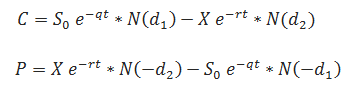where,

C is the price of the call option
P represents the price of a put option.
S0 is the underlying price,
X is the strike price,
σ represents volatility,
r is the continuously compounded risk-free interest rate,
t is the time to expiration, and
q is the continuously compounded dividend yield.
N(x) is the standard normal cumulative distribution function.

The formulas for d1 and d2 are given as: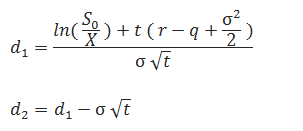To calculate the Greeks in options we use the Black-Scholes options pricing model.

Delta and Gamma are calculated as: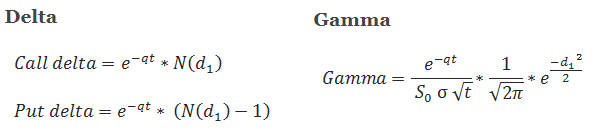In the example below, we have used the determinants of the BS model to compute the Greeks in options.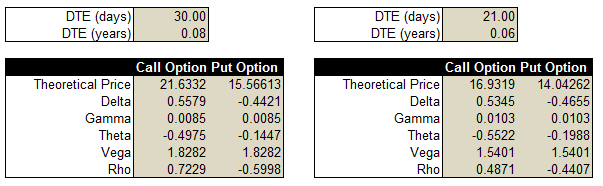At an underlying price of 1615.45, the price of a call option is 21.6332.

If we were to increase the price of the underlying by Rs. 1, the change in the price of the call, put and values of the Greeks in the option is as given below.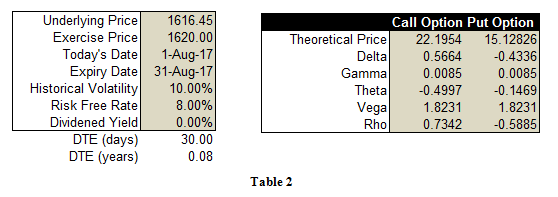As can be observed, the Delta of the call option in the first table was 0.5579. Hence, given the definition of the delta, we can expect the price of the call option to increase approximately by this value when the price of the underlying increases by Rs.1. The new price of the call option is 22.1954 which is

21.6332 + 0.5579 ~ 22.1954
22.1911 ~ 22.1954

Let’s move to Gamma, another Greek in option.

If you observe the value of Gamma in both the tables, it is the same for both call and put options contracts since it has the same formula for both options types. If you are going long on the options, then you would prefer having a higher gamma and if you are short, then you would be looking for a low gamma. Thus, if an options trader is having a net-long options position then he will aim to maximize the gamma, whereas in case of a net-short position he will try to minimize the gamma value.

The third Greek, Theta has different formulas for both call and put options. These are given below: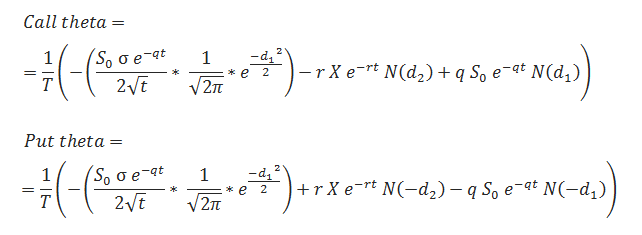In the first table on the LHS, there are 30 days remaining for the options contract to expire. We have a negative theta value of -0.4975 for a long call option position which means that the options trader is running against time.

He has to be sure about his analysis in order to profit from trade as time decay will affect this position. This impact of time decay is evident in the table on the RHS where the time left to expiry is now 21 days with other factors remaining the same. As a result, the value of the call option has fallen from 21.6332 to 16.9 behaviour 319. If an options trader wants to profit from the time decay property, he can sell options instead of going long which will result in a positive theta.

We have just discussed how some of the individual Greeks in options impact option pricing. However, it is very essential to understand the combined behaviour of Greeks in an options position to truly profit from your options position. If you want to work on options greeks in Excel, you can refer to this blog.

Let us now look at a Python package which is used to implement the Black Scholes Model.

### Python Library - Mibian

#### What is Mibian?

Mibian is an options pricing Python library implementing the Black-Scholes along with a couple other models for European options on currencies and stocks. In the context of this article, we are going to look at the Black-Scholes part of this library. Mibian is compatible with python 2.7 and 3.x. This library requires scipy to work properly.

#### How to use Mibian for BS Model?

The function which builds the Black-Scholes model in this library is the BS() function. The syntax for this function is as follows:

`BS([underlyingPrice, strikePrice, interestRate, daysToExpiration], volatility=x, callPrice=y, putPrice=z)`

The first input is a list containing the underlying price, strike price, interest rate and days to expiration. This list has to be specified each time the function is being called. Next, we input the volatility, if we are interested in computing the price of options and the option greeks. The BS function will only contain two arguments.

If we are interested in computing the implied volatility, we will not input the volatility but instead will input either the call price or the put price. In case we are interested in computing the put-call parity, we will enter both the put price and call price after the list. The value returned would be:

(call price + price of the bond worth the strike price at maturity) - (put price + underlying asset price)

The syntax for returning the various desired outputs are mentioned below along with the usage of the BS function. The syntax for BS function with the input as volatility along with the list storing underlying price, strike price, interest rate and days to expiration:

`c = mibian.BS([1.4565, 1.45, 1, 30], volatility=20)`

Attributes of the returned value from the above-mentioned BS function:

```c.callPrice       Returns the call price
c.putPrice    Returns the put price
c.callDelta         Returns the call delta
c.putDelta    Returns the put delta
c.callDelta2     Returns the call dual delta
c.putDelta2  Returns the put dual delta
c.callTheta      Returns the call theta
c.putTheta      Returns the put theta
c.callRho         Returns the call rho
c.putRho         Returns the put rho
c.vega             Returns the option vega
c.gamma         Returns the option gamma```

The syntax for BS function with the input as callPrice along with the list storing underlying price, strike price, interest rate and days to expiration:

`c = mibian.BS([1.4565, 1.45, 1, 30], callPrice=0.0359)`

Attributes of the returned value from the above-mentioned BS function:

`c.impliedVolatility Returns the implied volatility from the call price`

The syntax for BS function with the input as putPrice along with the list storing underlying price, strike price, interest rate and days to expiration:

`c = mibian.BS([1.4565, 1.45, 1, 30], putPrice=0.0306)`

Attributes of the returned value from the above-mentioned BS function:

`c.impliedVolatility Returns the implied volatility from the put price`

The syntax for BS function with the inputs as callPrice and putPrice along with the list storing underlying price, strike price, interest rate and days to expiration:

`c = mibian.BS([1.4565, 1.45, 1, 30], callPrice=0.0359, putPrice=0.0306)`

Attributes of the returned value from the above-mentioned BS function:

`c.putCallParity Returns the put-call parity`

While Black-Scholes is a relatively robust model, one of its shortcomings is its inability to predict the volatility smile. We will learn more about this as we move to the next pricing model.

### Derman Kani Model

The Derman Kani model was developed to overcome the long-standing issue with the Black Scholes model, which is the volatility smile. One of the underlying assumptions of Black Scholes model is that the underlying follows a random walk with constant volatility. However, on calculating the implied volatility for different strikes, it is seen that the volatility curve is not a constant straight line as we would expect, but instead has the shape of a smile. This curve of implied volatility against the strike price is known as the volatility smile.

If the Black Scholes model is correct, it would mean that the underlying follows a lognormal distribution and the implied volatility curve would have been flat, but a volatility smile indicates that traders are implicitly attributing a unique non-lognormal distribution to the underlying. This non-lognormal distribution can be attributed to the underlying following a modified random walk, in the sense that the volatility is not constant and changes with both stock price and time. In order to correctly value the options, we would need to know the exact form of the modified random walk.

The Derman Kani model shows how to take the implied volatilities as inputs to deduce the form of the underlying’s random walk. More specifically a unique binomial tree is extracted from the smile corresponding to the random walk of the underlying, this tree is called the implied tree. This tree can be used to value other derivatives whose prices are not readily available from the market - for example, it can be used in standard but illiquid European options, American options, and exotic options.

### What is the Heston Model?

Steven Heston provided a closed-form solution for the price of a European call option on an asset with stochastic volatility. This model was also developed to take into consideration the volatility smile, which could not be explained using the Black Scholes model.

The basic assumption of the Heston model is that volatility is a random variable. Therefore there are two random variables, one for the underlying and one for the volatility. Generally, when the variance of the underlying has been made stochastic, closed-form solutions will no longer exist.

But this is a major advantage of the Heston model, that closed-form solutions do exist for European plain vanilla options. This feature also makes calibration of the model feasible. If you are interested in learning about these models in more detail, you may go through the following research papers,

• Derman Kani Model - “The Volatility Smile and Its Implied Tree” by Emanuel Derman and Iraj Kani.
• Heston Model - “A Closed-Form Solution for Options with Stochastic Volatility with Applications to Bond and Currency Options”

So far, you have understood options trading and how to analyse an option as well as the pricing models used. Now, to apply this knowledge, you will need access to the markets, and this is where the role of a broker comes in.

## Opening an options trading account

### How to choose a broker for Options Trading?

Before we open an options trading account with a broker, let’s go over a few points to take into consideration when we choose a broker.

• Understand your aim when you tread the options trading waters, whether it is a way of hedging risk, as a speculative instrument, for income generation etc.
• Does the broker provide option evaluation tools of their own? It is always beneficial to have access to a plethora of tools when you are selecting the right option.
• Enquire the transaction costs or the commission charged by the broker as this will eat into your investment gains.
• Some brokers give access to research materials in various areas of the stock market. You can always check with the broker about access to research as well as subscriptions etc.
• Check the payment options provided by the broker to make sure it is compatible with your convenience.

### Searching for the right broker

Once the required background research is done, you can choose the right broker as per your need and convenience. In the global market, a list of the top brokers is provided below:

### List of Top International Brokers (Options Trading)

The list of top international options brokers is given below:

• Interactive Brokers (\$0.01 commission/share)

### List of Top Indian Brokers (Options Trading)

The list of top Indian options brokers is given below:

• Zerodha
• ICICI Direct
• HDFC Securites
• ShareKhan
• Kotak Securities
• Angel Broking
• Axis Direct

Great! Now we look at some options trading strategies which can be used in the real world.

There are quite a few options trading strategies which can be used in today’s trading landscape. One of the most popular options trading strategies is based on Spreads and Butterflies. Let’s look at them in detail.

Spreads or rather spread trading is simultaneously buying and selling the same option class but with different expiration date and strike price. Spread options trading is used to limit the risk but on the other hand, it also limits the reward for the person who indulges in spread trading.

Thus, if we are only interested in buying and selling call options of security, we will call it a call spread, and if it is only puts, then it will be called a put spread.

Depending on the changing factor, spreads can be categorised as:

• Horizontal Spread - Different expiration date, Same Strike price
• Vertical Spread -  Same Expiration date, Different Strike price
• Diagonal Spread - Different expiration date, Different Strike price

Remember that an option’s value is based on the underlying security (in this case, stock price). Thus, we can also distinguish an option spread on whether we want the price to go up (Bull spread) or go down (Bear spread).

In a bull call spread, we buy more than one option to offset the potential loss if the trade does not go our way.

Let’s try to understand this with the help of an example.
The following is a table of the available options for the same underlying stock and same expiry date: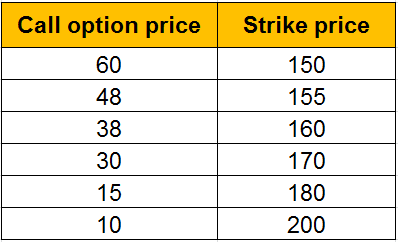Normally, if we have done the analysis and think that the stock can rise to \$200, one way would be to buy a call stock option with a strike price of \$180 for a premium of \$15. Thus, if we are right and the stock reaches \$200 on expiry, we buy it at the strike price of \$180 and pocket a profit of (\$20 -\$15) = \$5 since we paid the premium of \$15.

But if we were not right and the stock price reaches \$180 or less, we will not exercise the option resulting in a loss of the premium of \$15. One workaround is to buy a call option at \$180 and sell a call option for \$200 at \$10.

Thus, when the stock’s price reaches \$200 on expiry, we exercise the call option for a profit of \$5 (as seen above) and also pocket a profit of the premium of \$10 since it will not be exercised by the owner. Thus, in this way, the total profit is (\$5 + \$10) = \$15.

If the stock price goes above \$200 and the put option is exercised by the owner, the increase in the profit from bought call option at \$180 will be the same as the loss accumulated from the sold call option at \$200 and thus, the profit would always be \$15 no matter the increase in the stock price above \$200 at expiry date.

Let’s construct a table to understand the various scenarios.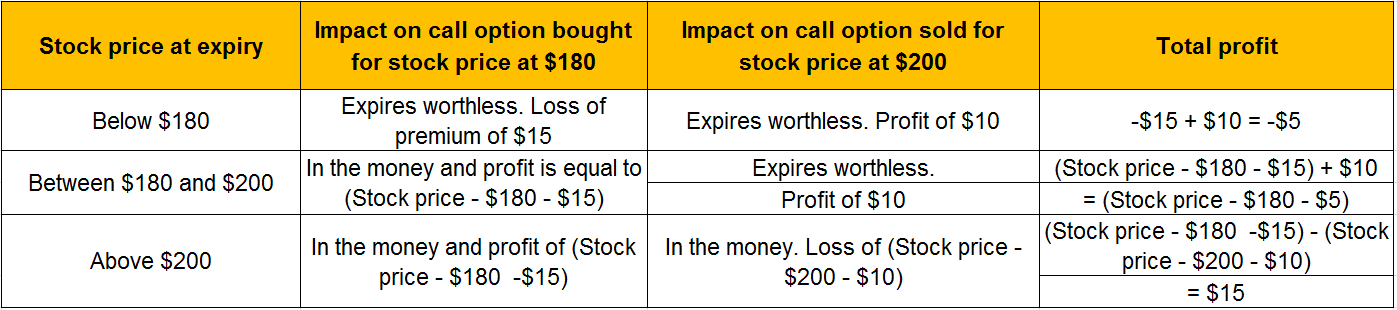You can go through this informative blog to understand how to implement it in Python.

The bull call spread was executed when we thought the stock would be increasing, but what if we analyse and find the stock price would decrease. In that case, we use the bear put spread.

Let’s assume that we are looking at the different strike prices of the same stock with the same expiry date.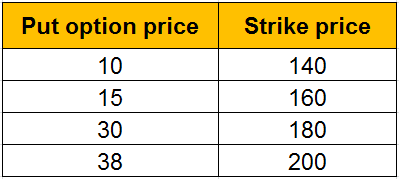One way to go about it is to buy the put option for the strike price of 160 at a premium of \$15 while selling a put option for the strike price of \$140 for the strike price of \$10.

Thus, we create a scenario table as follows: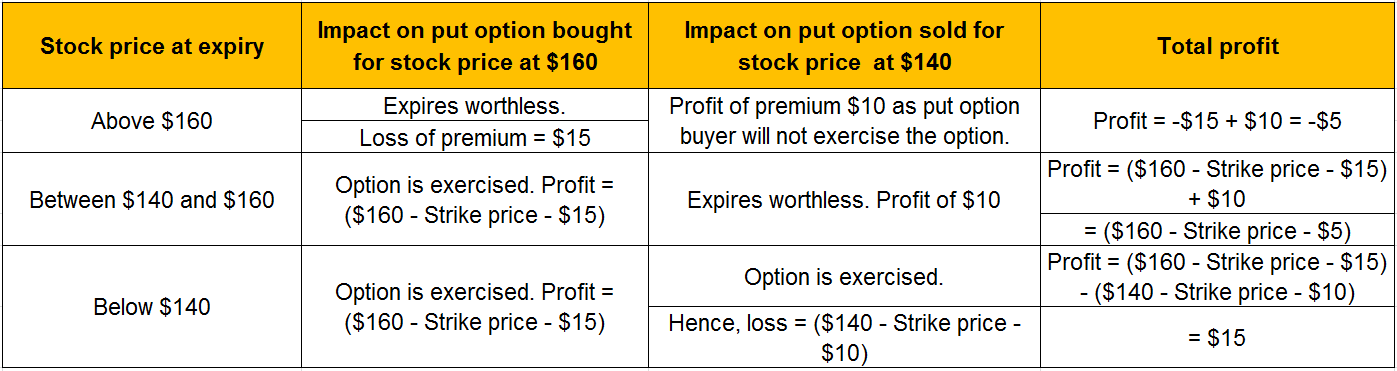In this way, we can minimize our losses by simultaneously buying and selling options. You can go through this informative blog to understand how to implement it in Python.

A butterfly spread is actually a combination of bull and bear spreads. One example of the Butterfly Options Strategy consists of a Body (the middle double option position) and Wings (2 opposite end positions).

• Its properties are listed as follows:
• It is a three-leg strategy
• Involves buying or selling of Call/Put options (unlike Covered Call Strategy where a stock is bought and an OTM call option is sold)
• Can be constructed using Calls or Puts
• 4 options contracts at the same expiry date
• Have the same underlying asset
• 3 different Strike Prices are involved (2 have the same strike price)
• Create 2 Trades with these calls